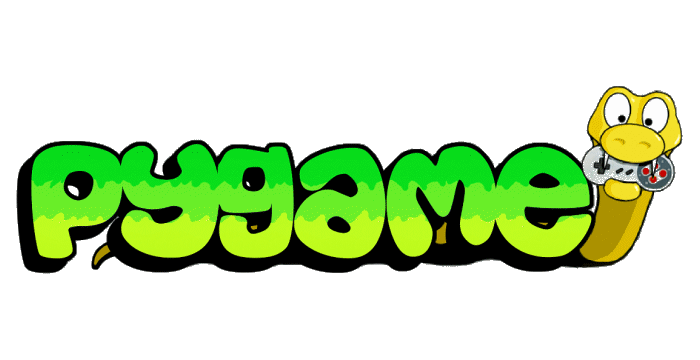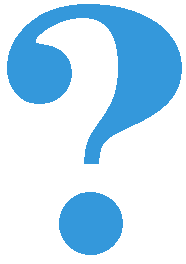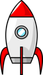# Obeleži sve kategorije koje odgovaraju problemu

### Još detalja - opišite nam problem

Uspešno ste prijavili problem!
Status problema i sve dodatne informacije možete pratiti klikom na link.
Nažalost nismo trenutno u mogućnosti da obradimo vaš zahtev.
Molimo vas da pokušate kasnije.

####Programming in Python, handbook

Acquiring information about keystrokes on a keyboard is very similar to reading the mouse buttons. Function pg.key.get_pressed() returns a tuple whose elements are used as logical values, showing for each key on the keyboard whether it is currently being pressed or not.

Because the keyboard has many more keys than the mouse, using indices 0, 1, 2, etc. for certain keys would be impractical. In order not to have to know which index in the tuple corresponds to which key, the PyGame library contains named constants that we use as indices. For example, constants pg.K_LEFT, pg.K_RIGHT, pg.K_UP, pg.K_DOWN correspond to the frequently used arrow keys. For the space key the corresponding constant is pg.K_SPACE, while for the letter keys, for example a, b, c the corresponding constants would be pg.K_a, pg.K_b, pg .K_c etc. The complete list of these constants can be seen here .

####Example - Spaceship: In this example, we have an image of a spaceship, which we move left and right in accordance with the pressed arrow keys. In addition, we can fire from the ship by pressing the space bar key.

First, pay attention to the highlighted part of the code with a lighter background (lines 23-37). That part is new here, and it is also commented in more detail in the code itself.

The rest of the program is just animating multiple objects, a technique known from before.So, after reading the status of all the keys and placing it in the tuple pressed, we can simply check the status of the keys we are interested in using if statement.

####Complete the following program so that the 4 arrow keys control the yellow circle, like in the example. The circle should not move if no arrows are pressed and move one pixel in the direction of the arrows that are pressed (opposite arrows cancel each other out).

####Complete the following program so that the arrows can control a ‘snake’ consisting of several squares, like in the example.

Variables d_row and d_col indicate the direction of movement of the snake. While no arrows are pressed, the value of these variables does not change and the snake continues to move in the same direction. Your task is to add commands for reading the status of the keyboard and calculating new values for (d_row, d_col) based on the arrows pressed, so that the movement continues in the chosen direction.

Hint: if the snake’s head was in the square (row, col), in the new frame it will be in the square (row + d_row, col + d_col). Check if you understood how you should assign values to the variables d_red, d_kol for each direction:

Q-76: If variables (d_row, d_col) are assigned values (0, -1), in which direction does the movement continue?

• Up
• No, values for up are (d_row, d_col) = (-1, 0)
• Down
• No, values for down are (d_row, d_col) = (1, 0)
• Left
• Correct
• Right
• No, values for right are (d_row, d_col) = (0, 1)

## Questions¶

As you answer the questions, go back to the “snake” program as needed and look up the parts you need to answer.

How many rows does the board have?

Q-77: What are the coordinates of the top left corner of the square (row, col)?

• x = row*a + a, y = col*a + a
• Try again
• x = col*a + a, y = row*a + a
• Try again
• x = row*a, y = col*a
• Try again
• x = col*a, y = row*a
• Correct

Q-78: Which sentences are true?

• In each frame list 'snake' is extended by a new element representing the new position of the snake's head.
• There is no such command in the program
• List 'snake' has the same number of elements throughout the program.
• Correct
• One element which represents the end of the snake's tail is removed from list 'snake' in each frame.
• There is no such command in the program